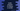# Kotlin example program to reverse a number# Introduction :

This tutorial will show you how to reverse a number in Kotlin. We will learn two different ways to do that. The first method will use one loop and the second method will use a different approach to solve it. This program will take the number as an input from the user, calculate the reverse and print it to the user.

## Method 1: Using a loop :

Create one new variable to hold the reverse number. Initialize it as 0. Using one loop, pick the rightmost digit of the number and add it to the reverse number. For example, if the given number is 123 :

1. Initialize the reverse number variable as 0
2. Pick 3 of 123 and convert it to 12. Add it to the reverse number. It will become 3.
3. Pick 2, the reverse number will be 32.
4. Pick 1, the reverse number will be 321.

### Kotlin program :

``````import java.util.Scanner

fun main(args: Array<string>) {
// 1
var num: Int
var reverse: Int = 0
val scanner = Scanner(System.`in`)

// 2
print("Enter a number : ")
num = scanner.nextInt()

// 3
while (num != 0) {
reverse = reverse * 10 + num % 10;
num /= 10;
}

// 4
println("Reverse number is : \$reverse")
}``````

### Explanation :

The commented numbers in the above program denote the step numbers below :

1. Create two integer variables. num is for holding the user input number and reverse is for holding the reverse number. the scanner is a Scanner variable to read the user input number. Don’t forget to import java.util.Scanner if you are using a Scanner.
2. Ask the user to enter a number. Read it using a scanner and store it in num.
3. Run one while loop. This loop will run until the value of num is not equal to zero. Inside the loop, we are updating the value of reverse. On each step, it takes the rightmost digit of num and adds it to reverse. We are also removing the rightmost digit and updating the value of num.
4. The loop will stop once the value of num is 0 i.e. all digits are added to reverse. Print the value of reverse to the user.

### Sample Output :

``````Enter a number : 8374847
Reverse number is : 7484738

Enter a number : 2233224
Reverse number is : 4223322

Enter a number : 1222345
Reverse number is : 54322211``````

## Method 2: Converting it to a string :

This is another way to reverse a number. Convert the number to a string and then reverse it. Kotlin provides toString() method to convert an integer to string and reversed() method to reverse a string. Note that the final reverse value will be string, not an integer.

``````import java.util.Scanner

fun main(args: Array<string>) {
var num: Int
var reverse: String
val scanner = Scanner(System.`in`)

print("Enter a number : ")
num = scanner.nextInt()

reverse = num.toString().reversed()

println("Reverse number is : \$reverse")
}``````

It will print outputs like below :

``````Enter a number : 12345
Reverse number is : 54321

Enter a number : 1009890
Reverse number is : 0989001``````

Note that with the first method, if you input any number with trailing zeros like 100, it will not print the zeroes at start for the reverse number, as it is an integer. For 100, it will print 1. But with the second method, it will print these zeroes as we are using string, not an integer.